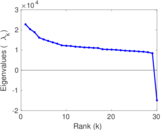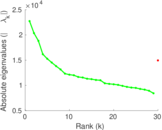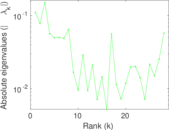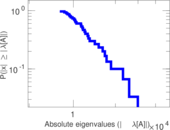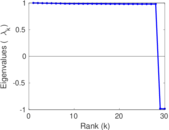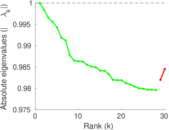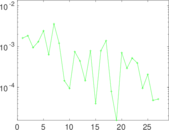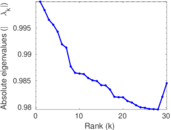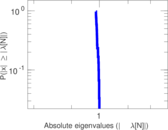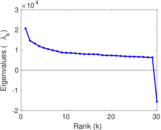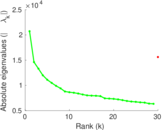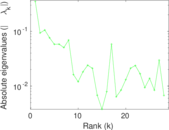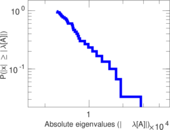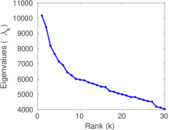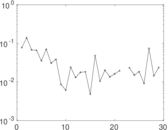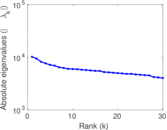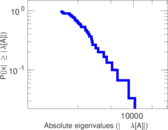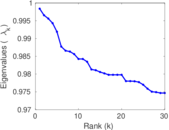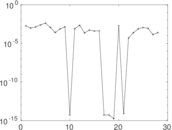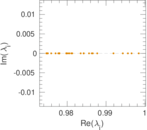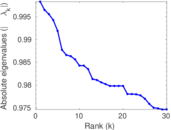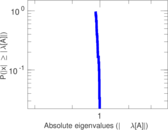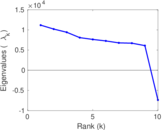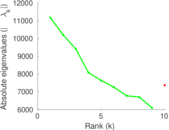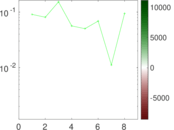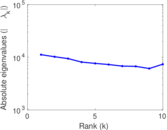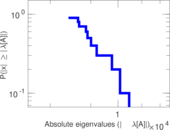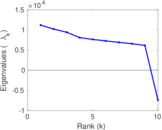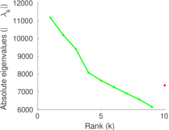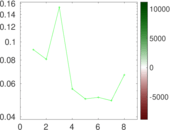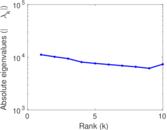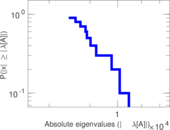Wikipedia talk (de)

This is the communication network of the German Wikipedia. Nodes represent users, and an edge from user A to user B denotes that user A wrote a message on the talk page of user B at a certain timestamp.

 Code Tde Internal name wiki_talk_de Name Wikipedia talk (de) Data source https://zenodo.org/record/49561 AvailabilityDataset is available for download Consistency checkDataset passed all tests Category Communication network Dataset timestamp 2017-10-27 Node meaning User Edge meaning Message Network format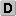Unipartite, directed Edge typeUnweighted, multiple edges Temporal dataEdges are annotated with timestamps ReciprocalContains reciprocal edges Directed cyclesContains directed cycles Loops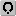Contains loops

Statistics

 Size n = 519,403 Volume m = 6,729,794 Unique edge count m̿ = 1,751,343 Loop count l = 2,174,035 Wedge count s = 22,215,138,984 Claw count z = 983,926,400,524,723 Cross count x = 3.502 66 × 1019 Triangle count t = 9,554,210 Square count q = 12,355,986,906 4-Tour count T4 = 187,711,464,036 Maximum degree dmax = 395,780 Maximum outdegree d+max = 395,774 Maximum indegree d−max = 27,165 Average degree d = 25.913 6 Fill p = 6.491 76 × 10−6 Average edge multiplicity m̃ = 3.842 65 Size of LCC N = 505,468 Size of LSCC Ns = 69,121 Relative size of LSCC Nrs = 0.133 078 Diameter δ = 13 50-Percentile effective diameter δ0.5 = 2.780 91 90-Percentile effective diameter δ0.9 = 3.746 41 Median distance δM = 3 Mean distance δm = 3.296 97 Gini coefficient G = 0.916 814 Balanced inequality ratio P = 0.092 355 7 Outdegree balanced inequality ratio P+ = 0.076 872 0 Indegree balanced inequality ratio P− = 0.137 522 Relative edge distribution entropy Her = 0.763 449 Power law exponent γ = 2.554 68 Tail power law exponent γt = 1.811 00 Tail power law exponent with p γ3 = 1.811 00 p-value p = 0.000 00 Outdegree tail power law exponent with p γ3,o = 1.741 00 Outdegree p-value po = 0.000 00 Indegree tail power law exponent with p γ3,i = 1.951 00 Indegree p-value pi = 0.000 00 Degree assortativity ρ = −0.124 769 Degree assortativity p-value pρ = 0.000 00 In/outdegree correlation ρ± = +0.723 786 Clustering coefficient c = 0.001 290 23 Directed clustering coefficient c± = 0.034 430 7 Spectral norm α = 22,742.4 Operator 2-norm ν = 18,161.3 Cyclic eigenvalue π = 10,171.3 Algebraic connectivity a = 0.033 983 0 Spectral separation |λ1[A] / λ2[A]| = 1.117 23 Reciprocity y = 0.223 920 Non-bipartivity bA = 0.342 587 Normalized non-bipartivity bN = 0.018 009 7 Algebraic non-bipartivity χ = 0.033 969 5 Spectral bipartite frustration bK = 0.001 349 57 Controllability C = 413,087 Relative controllability Cr = 0.795 311

Plots

Fruchterman–Reingold graph drawingDegree distribution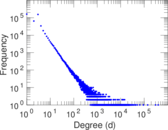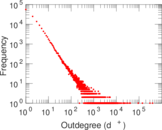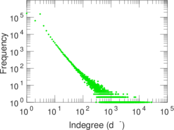Cumulative degree distribution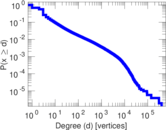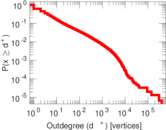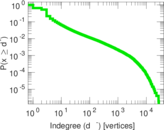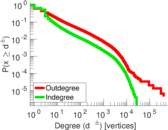Lorenz curve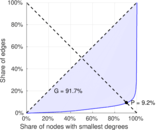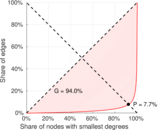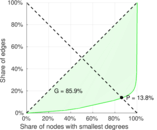Spectral distribution of the adjacency matrix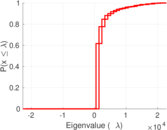Spectral distribution of the normalized adjacency matrix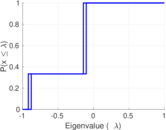Spectral distribution of the Laplacian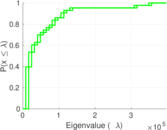Spectral graph drawing based on the adjacency matrix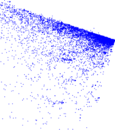Spectral graph drawing based on the Laplacian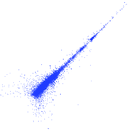Spectral graph drawing based on the normalized adjacency matrixDegree assortativity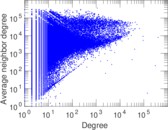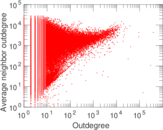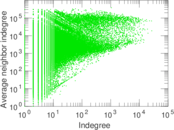Zipf plot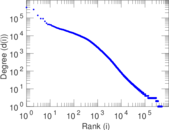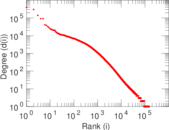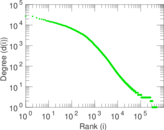Hop distribution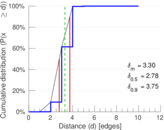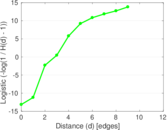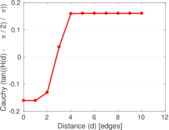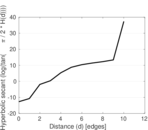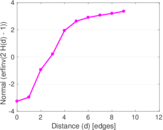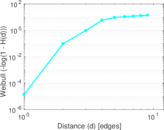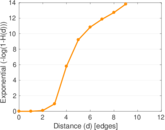In/outdegree scatter plot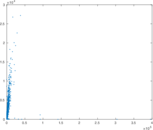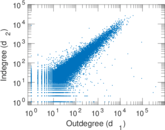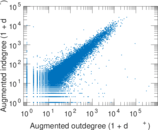Edge weight/multiplicity distribution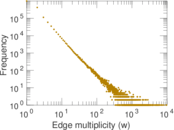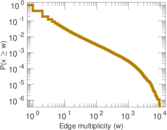Clustering coefficient distribution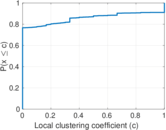Average neighbor degree distribution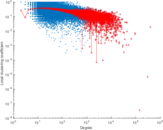Temporal distribution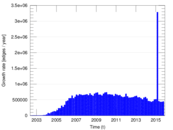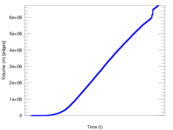Diameter/density evolution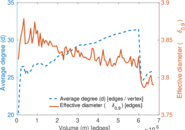SynGraphy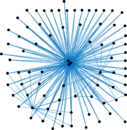Inter-event distribution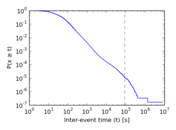Node-level inter-event distribution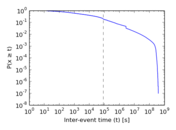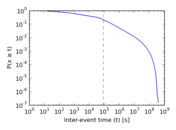Matrix decompositions plots